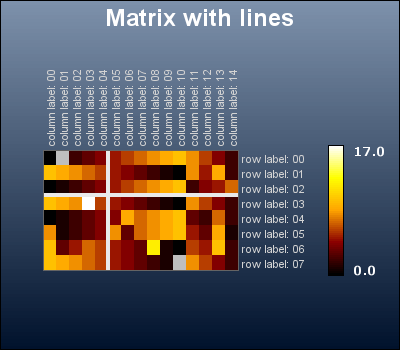## 22.6. Adding marker lines to the matrix plot

### Note

This feature was added in 3.1.1p

In order to mark important divisions in the plot it is possible to add an instance of `class PlotLine` (either horizontal or vertical line) to each plot in the graph. For example, to add one vertical and one horizontal plot line the following lines can be added to the matrix script

 ```1 2 3 4 5 6 7 8 9 10 11 12 13 14 15 ``` ```AddLine(\$l1); \$mp->AddLine(\$l2); // this could also be done as // \$mp->AddLine(array(\$l1,\$l2)); ... ?>```

The example in Figure 22.11 shows an example of this

Figure 22.11. Adding plot lines to the matrix plot `(matrix_ex06.php)`